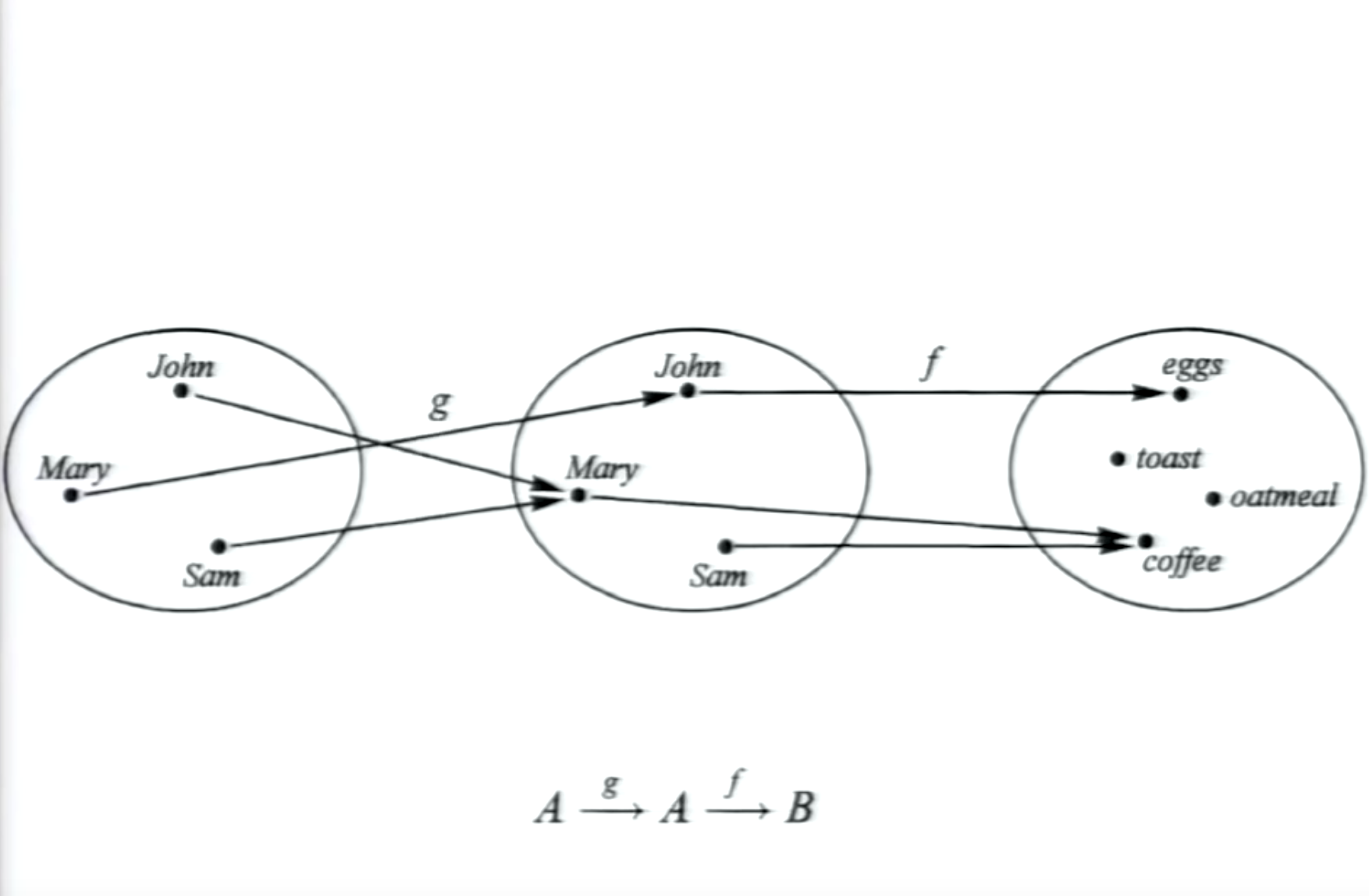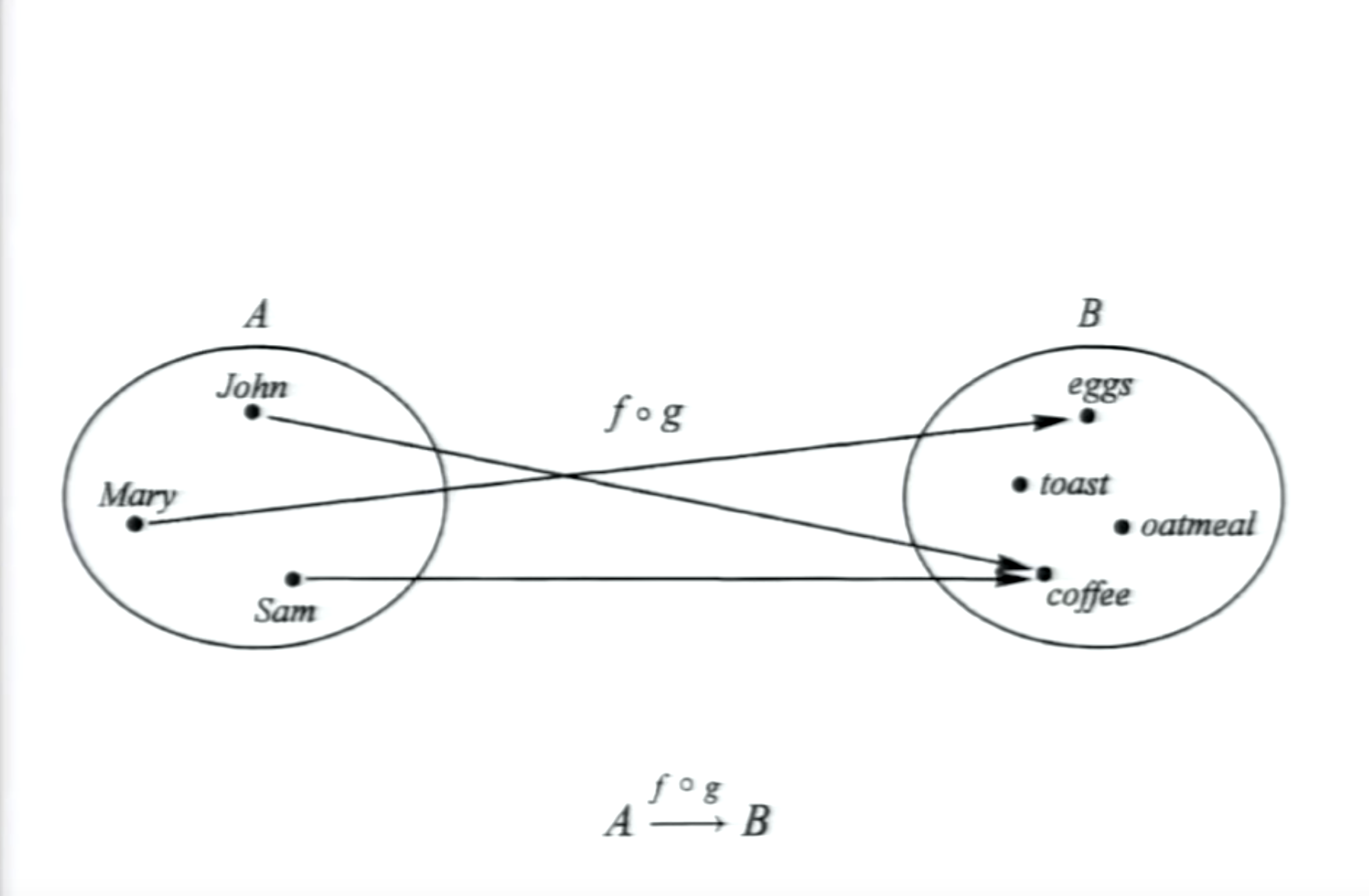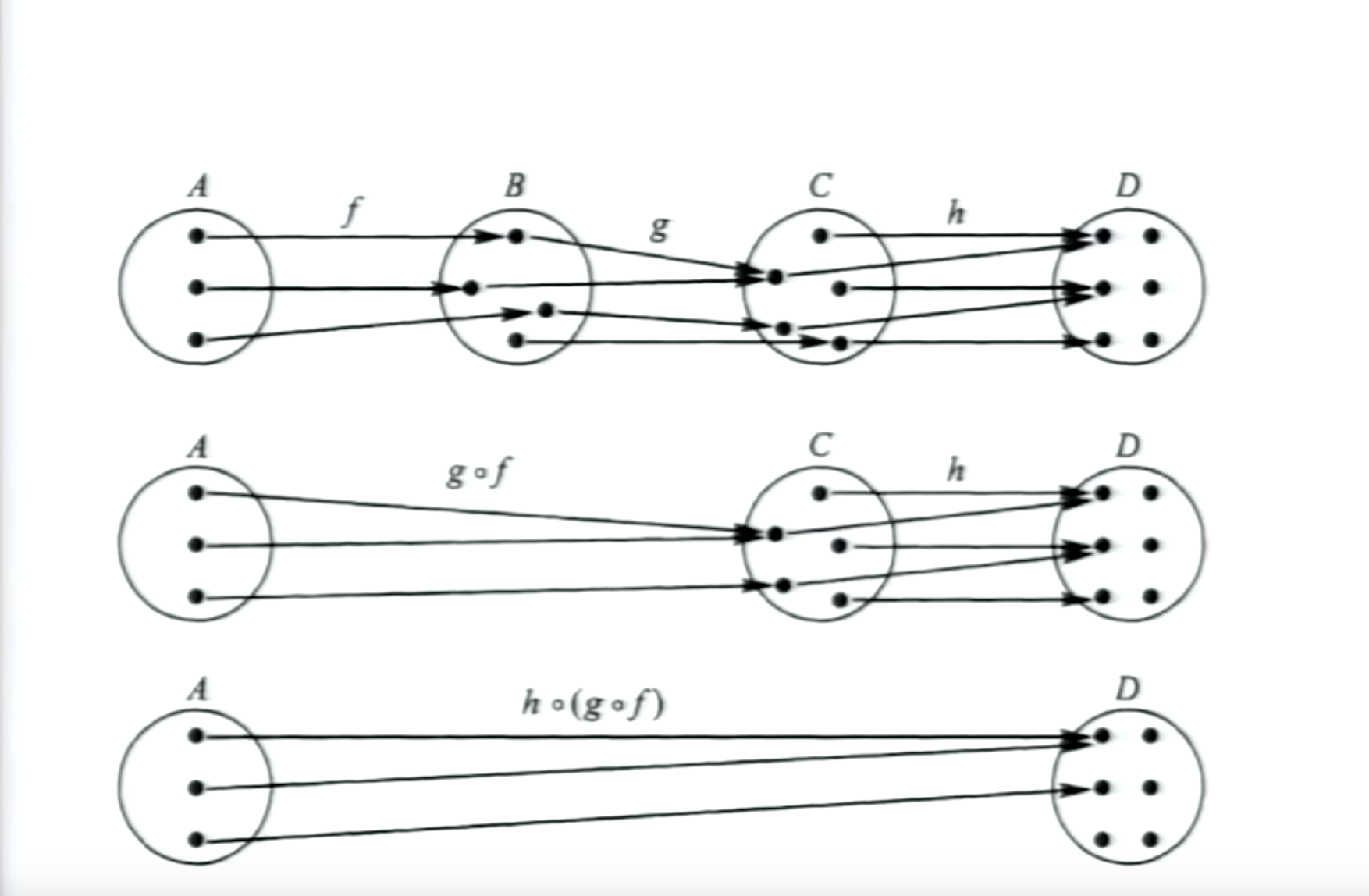## 柯里化(Curry)

```// add :: Num a => a -> a -> a
return function(y) {
return x + y
}
}

```

```// add3 :: Num a => a -> a
```

```Function.prototype.autoCurry = function() {
let func = this
return function curried(...args) {
if (args.length >= func.length) {
return func(...args)
} else {
return function (...args2) {
return curried(...args, ...args2)
}
}
}
}
```

```// fullname :: [Char] -> [Char] -> [Char] -> [Char]
let fullName = function(first, middle, last) {
return first + ' ' + middle + ' ' + last
}.autoCurry()
```

```fullName('Thomas', 'Jeffrey', 'Hanks') // Thomas Jeffrey Hanks
```

```let someThomas = fullName('Thomas')
someThomas('Alva', 'Edison') // Thomas Alva Edison
```

```let someThomasCruise = someThomas('Cruise')
someThomasCruise('Mapother') // Thomas Cruise Mapother
someThomasCruise('SomeOne') // Thomas Cruise SomeOne
```

```// modulo :: Integral a => a -> a -> a
let modulo = function (divisor, dividend) {
return dividend % divisor
}.autoCurry()
```

```modulo(3)(9) // 0
modulo(3, 9) // 0
```

```// isOdd :: Integral a => a -> a
let isOdd = modulo(2)
isOdd(6) // 0
isOdd(5) // 1
```

```// filter :: (a -> Bool) -> [a] -> [a]
let filter = function(f, xs) {
return xs.filter(f)
}.autoCurry()

filter(isOdd, [1,2,3,4,5]) // [1, 3, 5]
```

```let getTheOdds = filter(isOdd)
getTheOdds([1,2,3,4,5]) // [1, 3, 5]
```

`lodash` 是一个前端很广泛使用的函数库，但是它并不是真正的函数式，`Ramda` 则更函数式一点。我们来用这两个库来实现一个同样的函数：对于一个二维数组，返回一个新的二维数组，数组中每一项是原数据每一项的前两项。

lodash 的实现：

```// [[a]] -> [[a]]
function firstTwoLetters(words) {
return _.map(words, function(word) {
return _.take(word, 2)
})
}
firstTwoLetters([[1,2,3], [4,5,6]]) // [[1, 2], [4, 5]]
```

```function firstTwo(words) {
return _.map(words, _.take(2))
}
```

```let firstTwo = _.map(_.take(2))
```

```let firstTwo = R.map(R.take(2))
firstTwo([[1,2,3], [4,5,6]]) // [[1, 2], [4, 5]]
```

## 合成(Composition)

```// last :: [a] -> a
function last(xs) {
let sx = R.reverse(xs)
}

last([1,2,3]) // 3
```

compose 可以组合多个函数，将上一个函数的返回值作为下一个函数的参数。

```compose(f, g)(x) === f(g(x))
compose(f, g, h)(x) === f(g(h(x)))
```

```function compose(...funcs) {
return function(...args) {
let current = funcs.length - 1
let firstFunc = funcs[current]
let result = firstFunc.apply(this, args)

while(current--) {
result = funcs[current].call(this, result)
}

return result
}
}

```

```// wordCount :: [Char] -> Integral
function wordCount(str) {
let words = R.split(' ', str)
return R.length(words)
}

wordCount("I have four letters") // 4

let wordCount2 = compose(R.length, R.split(' '))
wordCount2("I have four letters") // 4
```